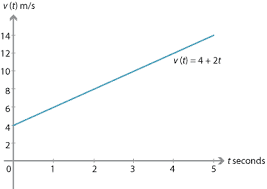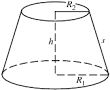## How to Calculate and Solve for Acceleration | MotionThe image above represents acceleration.

To compute for acceleration, two essential parameters are needed and these parameters are angular velocity (ω) and radius (r).

The formula for calculating acceleration:

a = ω²r

Where;

a = Acceleration
ω = Angular Velocity

Let’s solve an example;
Given that the angular velocity is 24 and the radius is 12. Find the acceleration?

This implies that;

ω = Angular Velocity = 24

a = ω²r
a = 24² x 12
a = 576 x 12
a = 6912

Therefore, the acceleration is 6912 m/s².

Calculating the Angular Velocity when the Acceleration and the Time is Given.

ω = √a / r

Where;

ω = Angular Velocity
a = Acceleration

Let’s solve an example;
Find the angular velocity when the acceleration is 50 with a radius of 2.

This implies that;

a = Acceleration = 50

ω = √a / r
ω = √50 / 2
ω = √25
ω = 5

Therefore, the angular velocity is 5.

## How to Calculate and Solve for the Height and Volume of a Conical Frustum | The Calculator EncyclopediaThe image above is a conical frustum.

To compute the volume of a conical frustum, three essential parameters are needed and this parameters are radius of the lower base (R), radius of the upper base (r) and height (h).

The formula for calculating the volume of a conical frustum:

V = πh(R² + Rr + r²)3

Where;
V = Volume of the conical frustum
R = Radius of the lower base
r = Radius of the upper base
h = Height of the conical frustum

Let’s solve an example;
Find the volume of the conical frustum when the lower base is 5 cm with an upper base of 9 cm and a height of 11 cm.

This implies that;
R = Radius of the lower base = 5 cm
r = Radius of the upper base = 9 cm
h = Height of the conical frustum = 11 cm

V = π(11)((5)² + (5)(9) + (9)²)3
V = (34.557)((25) + (45) + (81))3
V = (34.557)(151)3
V = 5218.1853
V = 1739.39

Therefore, the volume of the conical frustum is 1739.39 cm3.